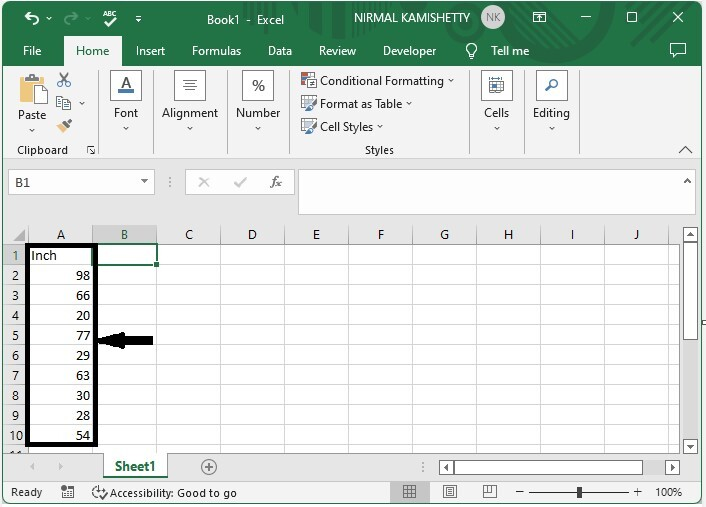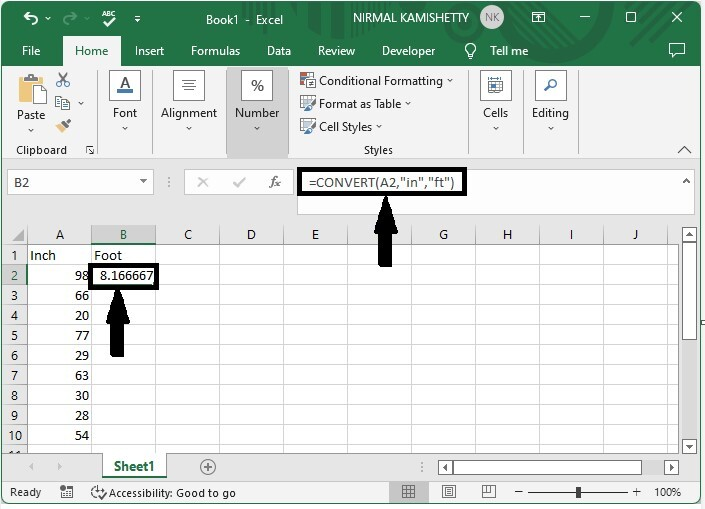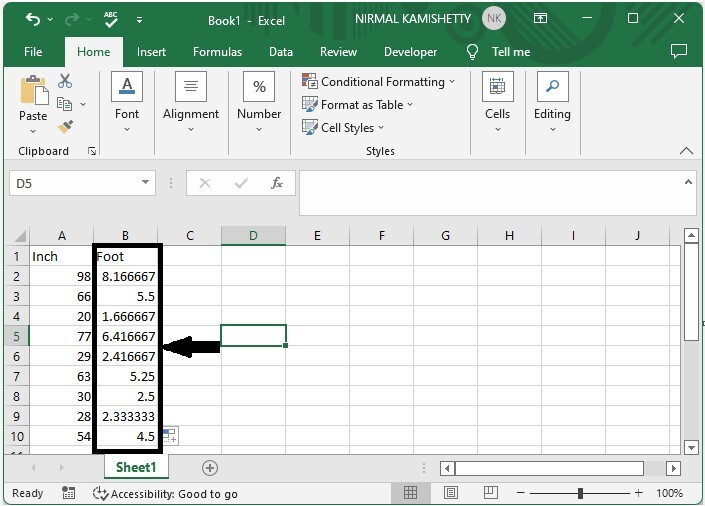# How to convert inch to foot, cm or mm in Excel?

There are several length measurement criteria, including meter, mile, inch, foot, and foot. The most commonly used metric units are the metre and the mile. When there are any values mentioned in inches, we can change them into multiple metric units.

In this tutorial, we will learn how to convert inches to feet, centimeters, and millimetres. A millimetre is one-tenth of a centimeter. We can convert inches to feet, cm, and mm directly using the formulas in Excel. Let us see how it is done.

## Converting inch to foot, cm or mm in Excel

Here we will use the CONVERT formula to get any one of the results, then use the auto-fill handle to get all the results. Let's look at a simple procedure for converting inches to feet, centimetres to millimetres, and more in Excel.

### Step 1

Consider an excel sheet with data that is similar to the data shown in the image below.To convert the values to feet, click on an empty cell, in this case cell B2, and enter the formula =CONVERT(A2,"in",”ft"), then press enter to get our first result, which looks like the image below.In the formula A2 is the address of our value on the sheet. in stands for inches and ft stands for feet. We can convert the values in to any metrics just by changing the names in formula/

### Step 2

Now to get all results drag down from the first result using the auto fill handle and our final result will be similar to below image.If we need to convert foot to Cm, we use the same process but the formula will change to =CONVERT(A2,”in”,”cm”) and for converting to mm we can use the formula as =CONVERT(A2,”in”,”mm”).

## Conclusion

In this tutorial, we used a simple example to show how you can use Excel to convert inches to feet, centimetres, and millimetres.